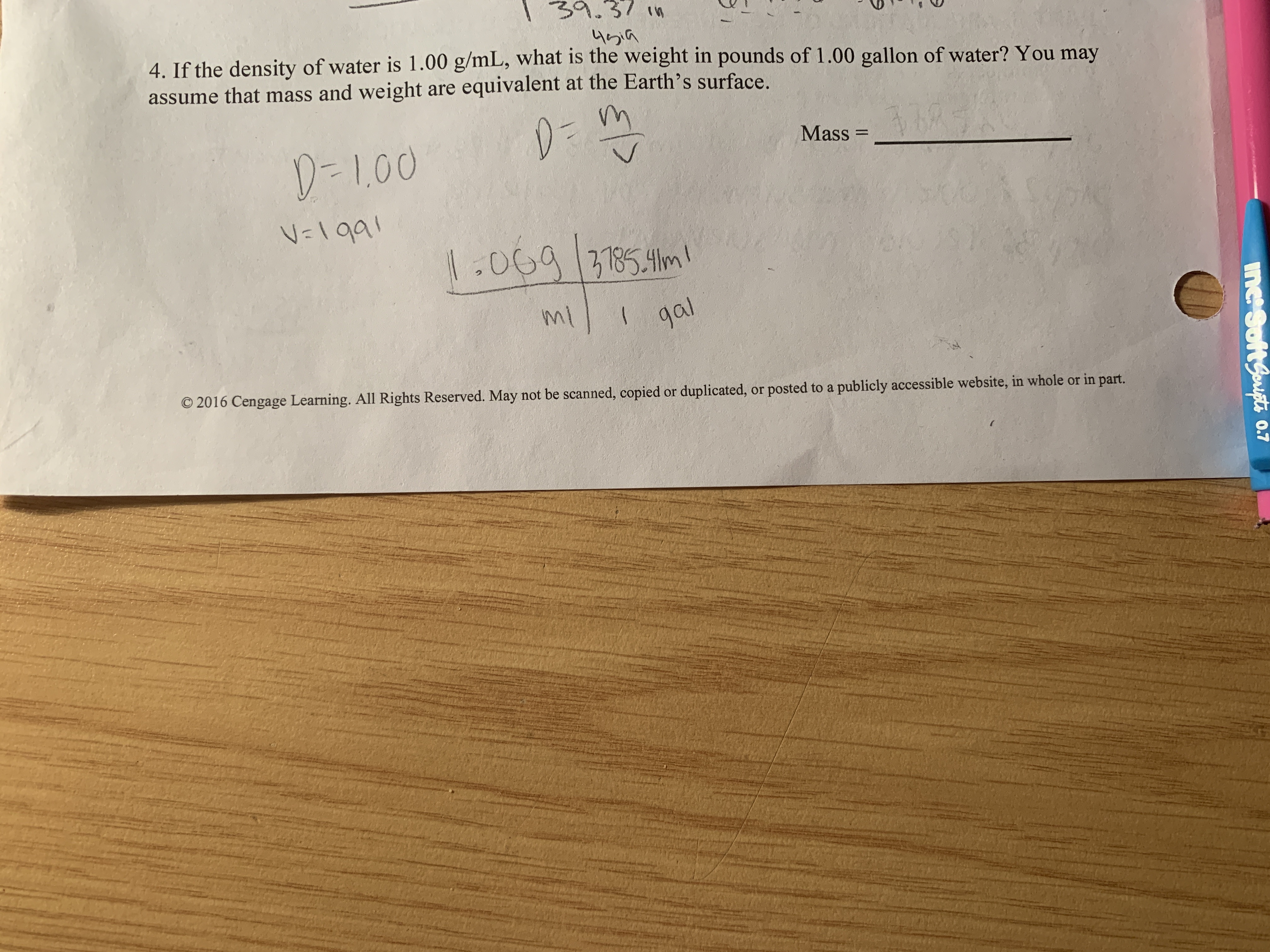# 4.If the density of water is 1.00 g/mL, what is the weight in pounds of 1.00 gallon of water? You mayassume that mass and weight are equivalent at the Earth's surface.Mass--1,00NTaamlgats Reserved. May not be scanned, copied or duplicated, or posted to a publicly accessible website, in whole or in part.

Question
1154 views

I am confused on whether I should take the amount in ml and put it back into the density formula. Honestly i might be reading too deep into the question.help_outlineImage Transcriptionclose4. If the density of water is 1.00 g/mL, what is the weight in pounds of 1.00 gallon of water? You may assume that mass and weight are equivalent at the Earth's surface. Mass- -1,00 NTaa mlga ts Reserved. May not be scanned, copied or duplicated, or posted to a publicly accessible website, in whole or in part. fullscreen
check_circle

star
star
star
star
star
1 Rating
Step 1

Density of water is equal to 1.00 g/ml.

density is related to the mass (g) and volume (ml) by the formula,

density (g/ml) = mass(g)/volume (ml)

since density of water = 1.00 g/ml

that is, 1.00 g.ml = mass(g)/volume(ml)

which makes, mass (g) of water = volume (ml) of water

Given here, volume of water = 1.00 gallon, which is equal to mass of water

Convert volume of water from gallon (volume) to pounds (mass)

conversion factor for gal...

### Want to see the full answer?

See Solution

#### Want to see this answer and more?

Solutions are written by subject experts who are available 24/7. Questions are typically answered within 1 hour.*

See Solution
*Response times may vary by subject and question.
Tagged in

### Chemistry